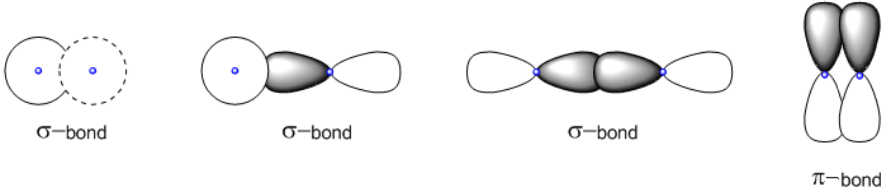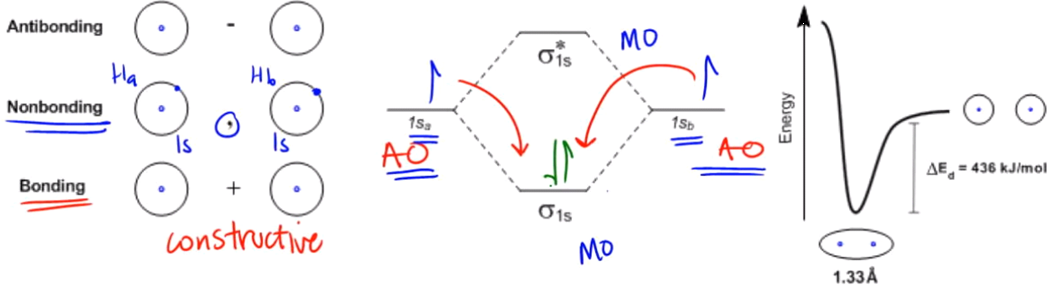Start typing, then use the up and down arrows to select an option from the list.# Organic Chemistry

Learn the toughest concepts covered in Organic Chemistry with step-by-step video tutorials and practice problems by world-class tutors.

1. A Review of General Chemistry

# Molecular Orbitals

When atomic orbitals interfere constructively with each other, they increase the chances of finding electrons between nuclei, forming what we call a bond.

## Types of Bonds

• A sigma (σ)-bond is formed by one region of constructive overlap between orbitals (regardless of the type of orbital used).
• A pi (π)-bond is formed by two regions of constructive overlap between orbitals (p-orbitals).1

#### concept

What’s the difference between sigma and pi bonds?2m
Play a video:
we were just talking about constructive and destructive interference, right? And we said that when things constructively interfere, they're gonna create unusual regions of shared electron density. That's just another way of me saying higher probability. Okay, called bonds. Okay, so remember that a bond is just an area where you wouldn't expect to find electrons. Usually, you would say there shouldn't be electrons here. According to the math, if I did the math, there should not be electrons. But for some reason, these orbital's are interfering with each other constructively, so they're increasing the chance of finding electrons there. Does that make sense? Cool. So it turns out that there's actually multiple ways to make these bonds or these shared regions of electron density. So let's talk about the simplest ways first, which is the Sigma bonds. So, as you see here, I have Sigma Sigma sigma. Alright, first of all you should know is that Sigma is going to be synonymous with the words single. Okay, so whenever I say ah, single bond, I'm always referring to a Sigma Bond. What that means is that a single a single bond is a region of one region of overlap okay. There's only one place where these orbital's air coming together, and they're making that bond happen. All right. It turns out that there's actually several ways to make this region of overlap, though there's several ways to make a single bond. We could have an s orbital and an s orbital. Okay? Or we could have an s orbital and a P orbital, or we could have a P orbital and a P orbital. Okay, The important part is that they all count a signal bonds as long as there's only one region of overlap. All right, so those are all the different types of signal bonds I could make. Now, another type of bond that I could make is one that has more than one region of overlap. All right. And that would be, for example, a pi bon. In fact, it has. It would have to regions of overlap from two p. Orbital's coming together. Okay, As you can see, there's a region at the top. There's a region at the bottom. Okay? Just you know, pi bonds are found in double bonds. Okay, so now I'm going to clarify this in a little bit pie bond and dull bond are not the same exact thing. But you should know is that a double bond has a pipe on in it. All right, cool.

## The LCAO Model:Predicting Molecular Orbitals

2

#### concept

What’s the difference between atomic and molecular orbitals?11m
Play a video:

The hexagon-thingy in the middle is the LCAO (Linear Combination of Atomic Orbitals):

• The side orbitals are your atomic orbitals (like you are used to drawing)
• The top and bottom orbitals represent atomic orbital overlap (molecular orbitals).
•  When atomic orbitals constructively interfere, they create bonding molecular orbitals that are more stable than the original atomic orbitals.## Bond Lengths and Strengths

3

#### concept

Sigma bond vs. pi bond, which is stronger?3m
Play a video: